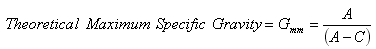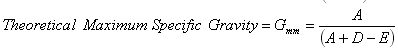# Theoretical Maximum Specific Gravity

## Overview

The theoretical maximum specific gravity (Gmm) of a HMA mixture is the specific gravity excluding air voids. Thus, theoretically, if all the air voids were eliminated from an HMA sample, the combined specific gravity of the remaining aggregate and asphalt binder would be the theoretical maximum specific gravity. Theoretical maximum specific gravity can be multiplied by the density of water (62.4 lb/ft3 or 1000 g/L) to obtain a theoretical maximum density (TMD) or “Rice” density (named after James Rice, who developed the test procedure).

Theoretical maximum specific gravity is a critical HMA characteristic because it is used to calculate percent air voids in compacted HMA. This calculation is used both in Superpave mix design and determination of in-place air voids in the field.

Theoretical maximum specific gravity is determined by taking a sample of loose HMA (i.e., not compacted), weighing it and then determining its volume by calculating the volume of water it displaces (Figure 1). Theoretical maximum specific gravity is then the sample weight divided by its volume.

The standard theoretical maximum specific gravity test is:

• AASHTO T 209 and ASTM D 2041: Theoretical Maximum Specific Gravity and Density of Bituminous Paving Mixtures

## Background

The theoretical maximum specific gravity test is integral to Superpave mix design as well as field quality assurance. Theoretical maximum specific gravity is used along with bulk specific gravity values from field cores and laboratory compacted specimens to calculate air voids and the in-place air voids of a HMA pavement. It is also used to calculate the amount of asphalt absorbed in a HMA mixture (Vba) , which is then used in determining the effective asphalt content (Pbe).

### Basic Premise

The basic premise of the maximum specific gravity is to divide the mass of the sample by the volume of the sample excluding the air voids. The mass is determined by measuring the dry mass of the sample either at the beginning of the test or after it has been dried at the end of the test. The volume is calculated by weighing the mass of the water displaced by the sample and dividing by the unit weight of water.

### In-place Density Measurement

As previously discussed, theoretical maximum specific gravity is needed to calculate air void content; therefore, it is involved in in-place air void determination during HMA pavement construction. In-place air void measurements are used as a measure of compaction (Figure 2). This is because compaction reduces the volume of air in HMA. Therefore, the characteristic of concern in compaction is the volume of air within the compacted HMA. This volume is typically quantified as a percentage of air voids by volume and expressed as “percent air voids”. Percent air voids is calculated by comparing a test specimen’s bulk specific gravity (Gmb) with its theoretical maximum specific gravity (Gmm) and assuming the difference is due to air. Once Gmm is known, portable non-destructive devices can be used to measure HMA density in-place. The terms “percent air voids” and “density” are often used interchangeably. Although this is not wrong, since density is used to calculate percent air voids, the fundamental parameter of concern is always percent air voids.

Percent air voids is typically calculated using Gmm and Gmb in the following equation:Each time density is to be determined a measure of bulk specific gravity is made by either coring the pavement and determining bulk specific gravity on the sample or using a non-destructive testing method. This bulk specific gravity is then compared to the most current theoretical maximum specific gravity to determine air voids. During HMA production and pavement construction, theoretical maximum specific gravity should be determined at regular intervals because it may change over time as the asphalt binder content and properties as well as aggregate properties vary over time.

If percent air voids is used as a primary quality assurance characteristic, there can be a tendency to control this characteristic at the expense of others. For instance, if adequate compaction is not being achieved, increasing asphalt binder content will fill more voids with asphalt binder and thus lower the air void content for the same amount of compaction. However, increased asphalt binder content can also potentially make a HMA mixture more likely to rut or shove.

### Relationship with Other Specific Gravities

Refer to Figure 3 for abbreviations.

1. The difference between Gmm and Gmb is volume. The weights are identical. The difference in volume is the volume of air in the compacted HMA mixture.
2. The following relationships are always true:
• Gmm ≥ Gmb
• Aggregate specific gravities (Gsb, Gsa, Gse and bulk SSD specific gravity ) are all ≥ Gmm

Figure 3: Typical weight-volume variables.

### Test Description

The following description is a brief summary of the test. It is not a complete procedure and should not be used to perform the test. The complete test procedure can be found in:

• AASHTO T 209 and ASTM D 2041: Theoretical Maximum Specific Gravity and Density of Bituminous Paving Mixtures

### Summary

A loose sample of either laboratory or plant produced HMA is weighed while dry (to determine its dry mass) and then a short procedure is used to determine the sample’s volume. The theoretical maximum specific gravity is then the sample’s mass divided by its volume.

### Approximate Test Time

45 minutes per test after samples are prepared (2 samples per test typically).

### Basic Procedure

Test samples may be representative of a mixture prepared in the laboratory or in a HMA plant. The mixture should be loose and broken up so that the fine aggregate is separated into particles smaller than 0.25 inches (6.25 mm) taking care not to fracture aggregate (Figure 4).

1. Place a loose sample at room temperature into a vacuum container and record the dry mass. If Weighing in Water is chosen in step 5, glass, plastic or metal bowls (Figure 5) as well as thick-walled flasks or vacuum desiccators are used. If Weighing in Air is chosen in step 5, flasks (Figure 6) or pycnometers are used.
2. Completely cover the sample by adding water at approximately 77°F (25°C) to the container.
3. Remove entrapped air in the sample by applying a vacuum of 27.75 mm Hg (3.7 kPa) to the pycnometer or flask for 15 minutes. The container should be agitated continuously by mechanical means (Video 1) or shaken vigorously by hand every two minutes.
4. Slowly release the vacuum.
5. Weigh the sample in water or air:
• Weighing in water. Suspend the container (which is filled with the sample and water) in a water bath at 77°F (25°C) for 10 minutes and record the mass.
• Weighing in air. Fill the container completely with water at 77°F (25°C). Determine the mass of the completely filled container within 10 minutes of releasing the vacuum.
In highly absorptive aggregate, water may seep in between the absorbed asphalt and the aggregate particle resulting in an erroneous dry weight measurement.To determine whether significant seepage has occurred, decant the sample through a towel (so that the fines are retained) held over the top of the container. Take several of the larger pieces of aggregate and break them. Examine the broken faces for wetness. Wetness indicates seepage. If seepage is detected, a supplemental procedure needs to be run on the sample at the end of the test. Generally, if the aggregate has a water absorption of less than 1.5 percent the supplemental procedure is not needed.
This procedure is accomplished by spreading the wet sample in front of a fan and weighing at 15 minute intervals. When the mass loss between weighings is less than 0.05 percent, the sample is said to be dry. This dry mass should be used for calculations. This is often called a “dry-back” procedure.
http://youtu.be/nQh6hotdOmEVideo 1: Mechanical agitation.

## Results

### Parameters Measure

Maximum specific gravity.

### Specifications

There is no specification for theoretical maximum specific gravity, but it is used to calculate other specified parameters such as air voids (Va) in laboratory compacted mixtures and in-place density in the field.

### Typical Values

Typical values for theoretical maximum specific gravity range from approximately 2.400 to 2.700 depending on the aggregate specific gravity and asphalt binder content. Unusually light or heavy aggregates may result in a value outside this typical range.

### Calculations (Interactive Equation)

Calculate and report Gmm to the nearest thousandth.

#### Weighing in Water MethodWhere:

• A = sample mass in air (g)
• C =mass of water displaced by the sample (g)

#### Weighing in Air MethodWhere:

• A = sample mass in air (g)
• D = mass of flask filled with water (g)
• E = mass of flask and sample filled with water (g)